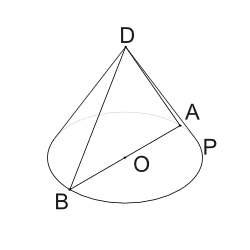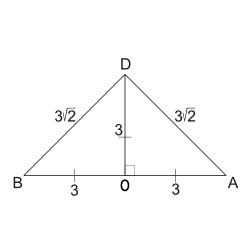We cover every section of the GMAT with in-depth lessons, 5000+ practice questions and realistic practice tests.

## Up to 90+ points GMAT score improvement guarantee

### The best guarantee you’ll find

Our Premium and Ultimate plans guarantee up to 90+ points score increase or your money back.

## Master each section of the test

### Comprehensive GMAT prep

We cover every section of the GMAT with in-depth lessons, 5000+ practice questions and realistic practice tests.

## Schedule-free studying

### Learn on the go

Study whenever and wherever you want with our iOS and Android mobile apps.

## The most effective way to study

### Personalized GMAT prep, just for you!Circle $$P$$ is the base of a right circular cone whose apex is $$D$$. $$AB$$ is a diameter of $$P$$. If $$\angle ADB$$ is a right angle and $$AD=3\sqrt{2}$$, then what is the volume of the cone?
Correct. [[snippet]] The cone is a right cone, so $$AD=DB$$. Since $$\angle ADB$$ is a right angle, $$\triangle ADB$$ is a right isosceles triangle. The ratio of the sides in a right isosceles triangle, a 45º-45º-90º triangle, is $$x{:}x{:}x\sqrt{2}$$, so if the legs are of length $$3\sqrt{2}$$, the hypotenuse $$AB$$, which is also the diameter of the base of the cone, is $$3\sqrt{2}\cdot\sqrt{2}=3\cdot2=6$$. To calculate the height of the cone, connect points $$D$$ and $$O$$. If radius $$OA$$ is half of the diameter, or 3, and $$DA=3\sqrt{2}$$, then triangle $$DAO$$ is also a right isosceles triangle. Thus, $$DO=OA=3$$.Now you can find the volume of the cone: > $$\displaystyle{ \frac{\pi r^2 h}{3}=\frac{\pi(9)(3)}{3}=9 \pi}$$.
$$3 \pi$$
$$6 \pi$$
$$9 \pi$$
$$18 \pi$$
$$27 \pi$$